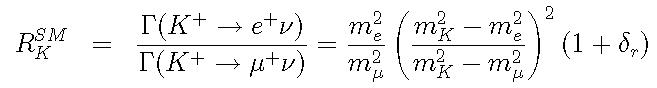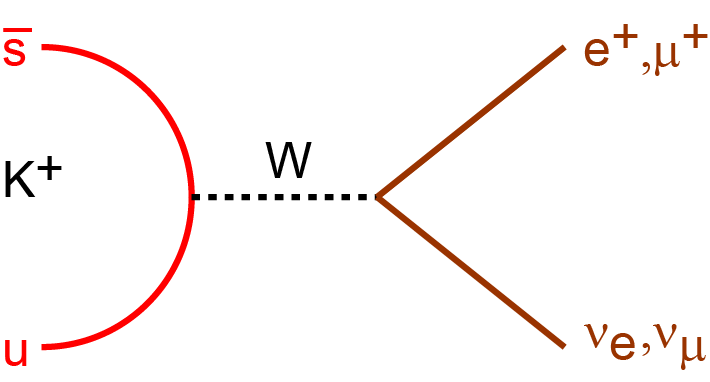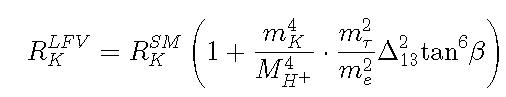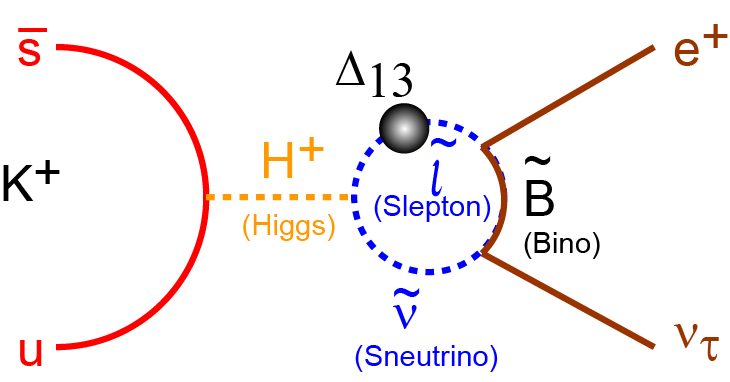﻿

Precise Measurement of Γ (K+→ e+ν)/Γ(K+→ μ+ν) using Stopped Positive Kaons

Home > Aim of our experiment > Physics motivation for RK

Physics motivation for RK ---Lepton universality tested in Kl2 and search for new physics beyond the SM

Abstract

We propose a precise measurement ratio of the decay width RK= Γ (K+→ e+ν)/Γ(K+→ μ+ν) to test lepton universality using a sub-system of the TREK experiment during low-intensity period. The Standard Model (SM) prediction for the RK value is highly precise (ΔRK/RK = 0.4×10-3) and a deviation from this value would very clearly indicate the existence of New Physics beyond the SM. Recently, a minimal supersymmetric (SUSY) extension of the SM (MSSM) has been considered as a candidate for the new physics to be tested by RK. A possible mechanism to detect the SUSY Lepton Flavor Violation effect through a deviation from the lepton universality has been discussed. The non-vanishing e-τ lepton mixing can change the RK value from the SM prediction at the percent level. In this proposed experiment, we aim at achieving an uncertainty for RK of better than ΔRK/RK = 2.5×10-3, which is about half of the current world record.

Physics Motivation for RK measurement

High precision electroweak tests represent a powerful tool to prove the Standard Model (SM) and to obtain indirect hints of new physics. The K+ → l+ν decay (Kl2), which is the simplest semileptonic decay among the K+ decay channels, is one of the best channels to perform such tests. Lepton universality, which is expressed as the identical coupling constant of the three lepton generations -- the electron, muon, and tau-- is a basic assumption in the SM. Violation of lepton universality clearly indicates the existence of other physics beyond the SM. The Kl2 decay diagrams are shown in the figure. This Kl2 hadronic form factor can be canceled out by forming the ratio of the electric (Ke2) and muonic (Kμ2) decay modes as,under the assumption of μ-e universality.The factor (me/mμ)2 accounts for the helicity suppression of Ke2 decay due to the V - A structure of the charged weak current, and, in other words, enhances the sensitivity to effects beyond the SM. As a result, the SM prediction of RSMK is known with excellent accuracy (ΔRK/RK = 0.4 × 10-3) and this makes it possible to search for new physics effects by a precise measurement of RK.Feynman diagram of Kl2 decay. The hadronic form factor fK is canceled out by forming the ratio of the decay widths. The SM prediction of RK is known with excellent accuracy.

Contribution from MSSM

Recently, in the kaon physics field, a minimal SUSY extension of the SM (MSSM) with R parity has also been considered as a candidate for new physics to be tested by RK. It is well known that models containing at least two Higgs doublets generally allow flavor violating couplings of the Higgs bosons with the fermions. In the MSSM, such Lepton Flavor Violation (LFV) couplings are absent at tree level. However, once non holomorphic terms are generated by loops and given a source of LFV among the sleptons, Higgs-mediated Hll LFV couplings are unavoidable. These effects have been widely discussed in the recent literature through the study of several processes, namely τ → lj lklk , τ → μη , μ-e conversion in nuclei, B → ljτ, H → lj lk , and li → ljγ. In the case of Kl2, in addition to the normal W± exchange shown in the upper figure, a possible mechanism to detect the LFV SUSY effect through a deviation from the μ-e universality has been discussed. A charged Higgs-mediated SUSY LFV contribution, as shown in the right figure, can be strongly enhanced by emitting a τ neutrino. This nonvanishing e-τ lepton mixing effect can be described as,where MH is the mass of the charged Higgs. The second term with Δ13 is a vertex correction due to the exchange of a Bino and a slepton, which represents the contribution of LFV effect generated from the off-diagonal flavor changing entries of the slepton mass matrix. A large enhancement factor m2τ /m2e can produce a sizable effect in RK through change of the Ke2 width.
.Contribution from charged Higgs-mediated LFV effect arising in SUSY model. Effect from non-vanishing e-τ mixing can be strongly enhanced by emitting a τ neutrino.

Contribution from other models

A precise measurement of the RK value can set constraints in certain other nonstandard model scenarios. RK constrains neutrino mixing parameters within SM extension involving sterile neutrino and/or 4th generation of quarks leptons. Scalar couplings arising from physics beyond the SM will also induce pseudoscalar interactions through loop corrections. In the case of R-parity violating SUSY, the exchange of various generations of squarks may lead to a nonuniversal contribution that results in a comparable deviation, and the measurement places substantial constraints on the possible size of R-parity violating effects

Other experimental studies

Recently, the NA48 and KLOE groups performed measurements. In the NA62 measurement, an in- flight kaon beam with particle momentum from 15 to 65 GeV/c, was adopted, and Kμ2 decay was the main background source in the Ke2 sample. The treatment of these backgrounds was one of the most difficult points to determine the RK value in the NA62 experiment. Since low energy kaons from φ → K+K- were used in the KLOE experiment, the kinematical resolution of Kl2 decays were much better than that in NA62. However, the experimental result was dominated by the statistical error because of low-intensity of the kaon beam. The NA62 and KLOE results were obtained to be
RK =(2.488 ± 0.007 ± 0.007) × 10-5(NA62) ,
RK =(2.493 ± 0.025 ± 0.019) × 10-5(KLOE) .
The combined result was determined by calculating the error-weighted averages as,
RK =(2.488 ± 0.009)×10-5(combined) ,
which is consistent with the SM within the error. Since the experimental error size is about 10 times larger than the theoretical one, there is still room for further improvement of the RK determination.

NA48/2 and NA62 at CERN
KLOE at Dafne